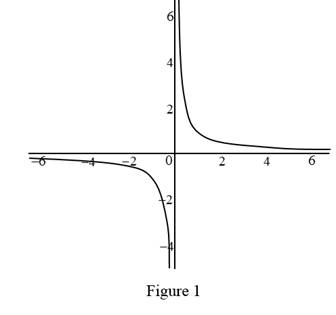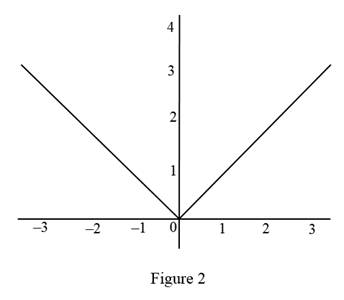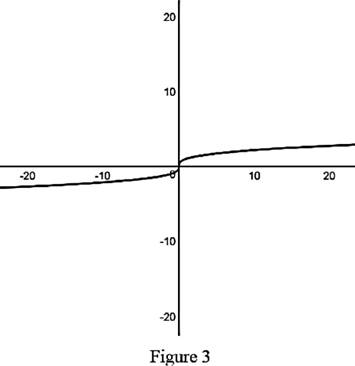# The function can fail to be differentiable.### Single Variable Calculus: Concepts...

4th Edition
James Stewart
Publisher: Cengage Learning
ISBN: 9781337687805### Single Variable Calculus: Concepts...

4th Edition
James Stewart
Publisher: Cengage Learning
ISBN: 9781337687805

#### Solutions

Chapter 2, Problem 15RCC
To determine

## To describe: The function can fail to be differentiable.

Expert Solution

### Explanation of Solution

The three main ways that the functions to be differentiable are given below:

(i) The function may be discontinuous at a point.

Example: Let the function f(x)=1x.

Graph:(ii) The function may have a corner at a point.

Example: Let the function f(x)=|x|.

Graph:(iii) The function may have a vertical tangent at a point.

Example: Let the function f(x)=|x|13|x|x has a vertical tangent line at x=0

Graph:### Have a homework question?

Subscribe to bartleby learn! Ask subject matter experts 30 homework questions each month. Plus, you’ll have access to millions of step-by-step textbook answers!